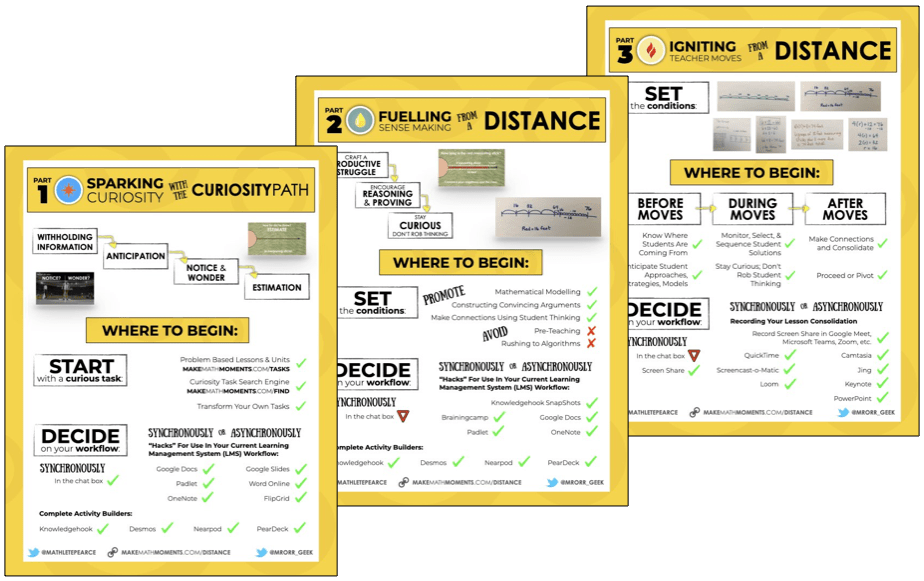# Visualizing Integer Division: Positive Divided By a Negative

In this Math Is Visual video animation and visual prompts, we will explore negative divided by a positive integer division conceptually through concrete and visual representations! We will also tackle this from two approaches: quotative division and partitive division!

So far in the integer division series, we have tackled the following ideas:

If you have yet to view those posts, you should definitely go back prior to moving on to ensure that you are ready to grasp our last step in the Division of Integers Series: Positive Number Divided By a Negative Number.

In this video, we will address the division of a positive dividend by a negative divisor first using partitive (or fair share) division, then quotative (or measured) division. The viewer will also be prompted to pause the video and try one their own using concrete manipualtives and/or visuals.

See the instructional guide below aimed to help teachers and/or parents use this activity with their own students/children.

## Visual Prompt #1: Partitive Division

In the video above, we start with dividing a negative by a negative through partitive division:

8 ÷ (-2) = ___

in words:

8 divided into -2 groups gives a result of ___ per group

So, let’s have the viewer model concretely and/or visually the following: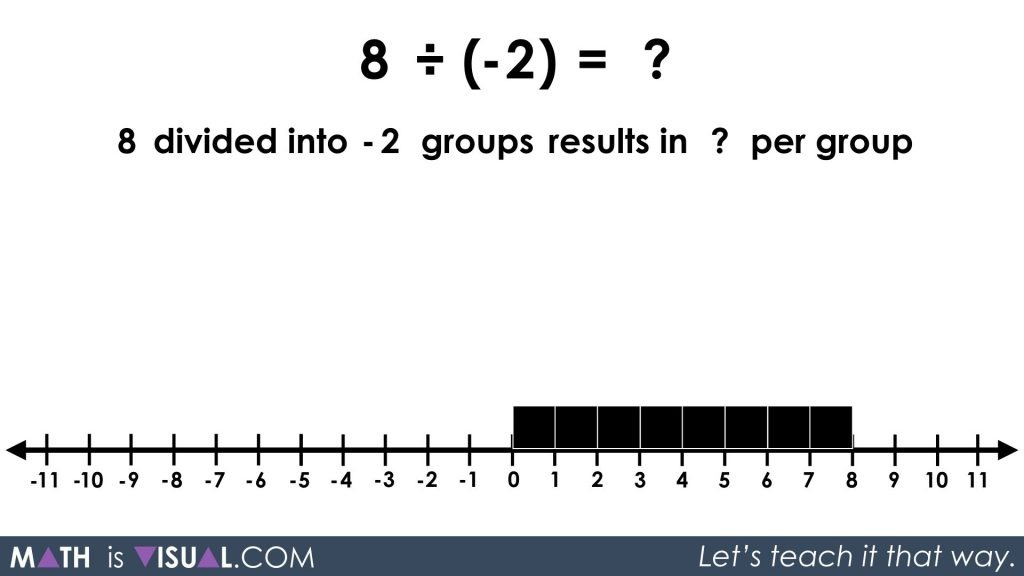We can approach this using a set or linear model where we are spatially splitting the quantity into 2 equal “negatively charged” groups and counting the number of objects in each group. We can think of this as repeated subtraction if we were to fair share 1 object at a time or 2 objects at a time, up to a quantity that a student is comfortable to subitize quickly for distributing equally to the two groups.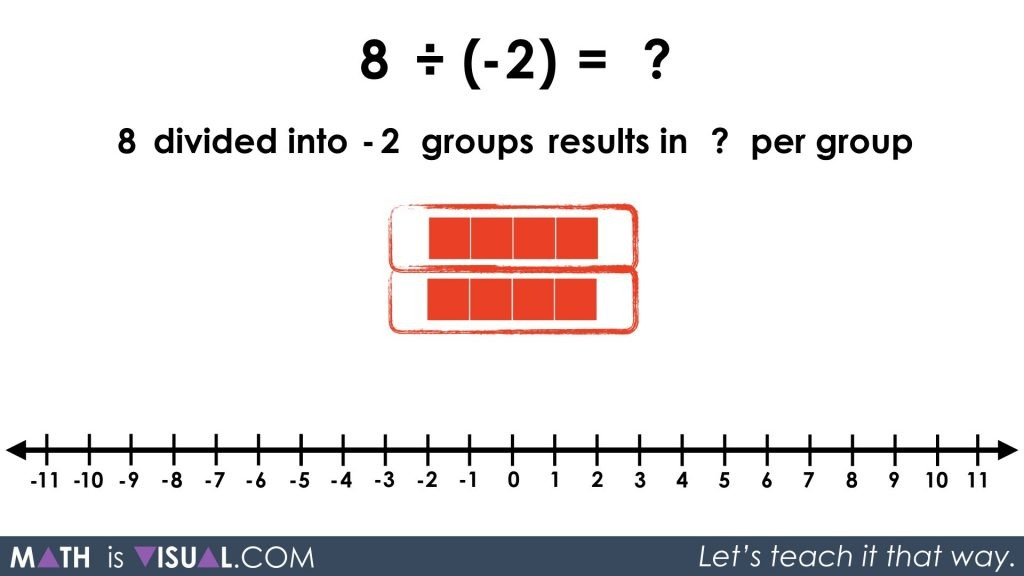## Visual Prompt #2: Quotative Division

Now we move on to approaching the same problem from a partitive division perspective:

8 ÷ (-2) = ____

or

8 divided into groups of -2 gives a result of ____ groups.The problem we run into here is that we want to determine how many groups of -2 we can create from 8.

This is a problem because we can’t make any groups of -2 (or so it may seem).

If we determine first how many groups of +2 we can make (4 in total), we can negate the negative of each group by using negatively charged groups: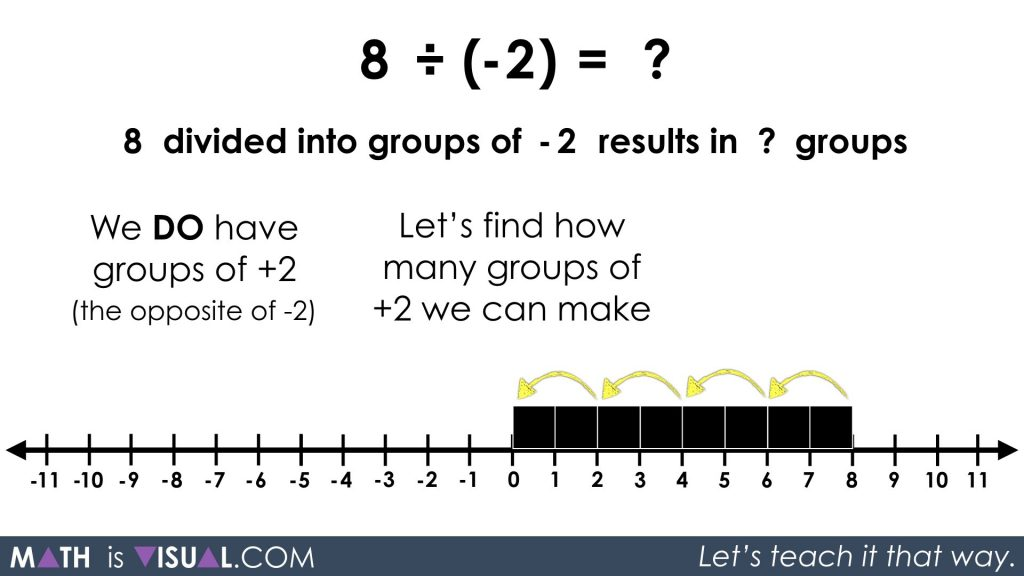So instead of having 4 positively charged groups of +2, we can express those groups as 4 negatively charged groups of -2.

In other words, we have:

-4 groups of -2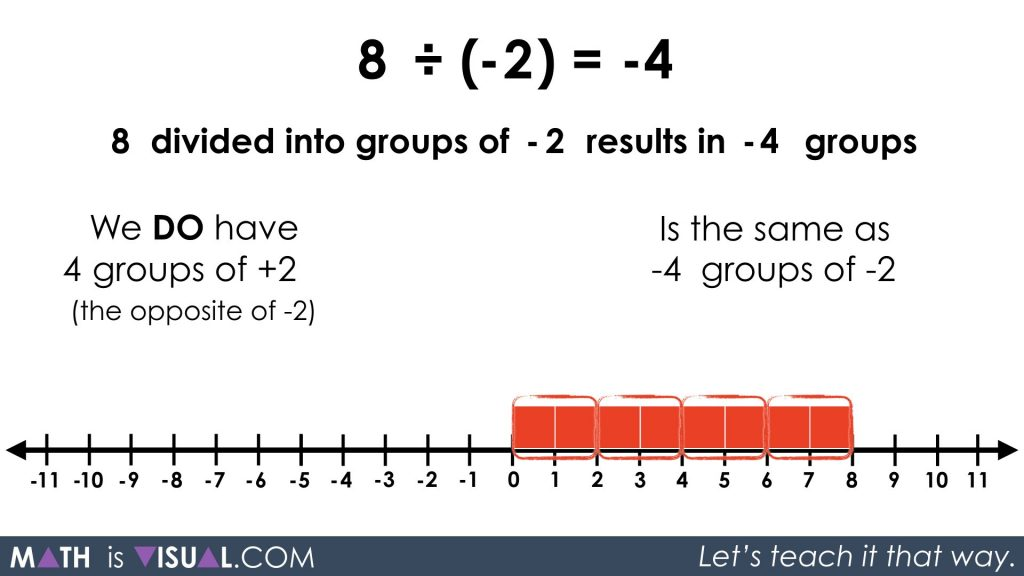### Other possible representations and strategies:

Consider determining how many groups of +2 we can “remove” since division represents repeated subtraction. Once we find out how many groups of +2 we can remove, we can then express those groupings negatively to create negative values in the groups: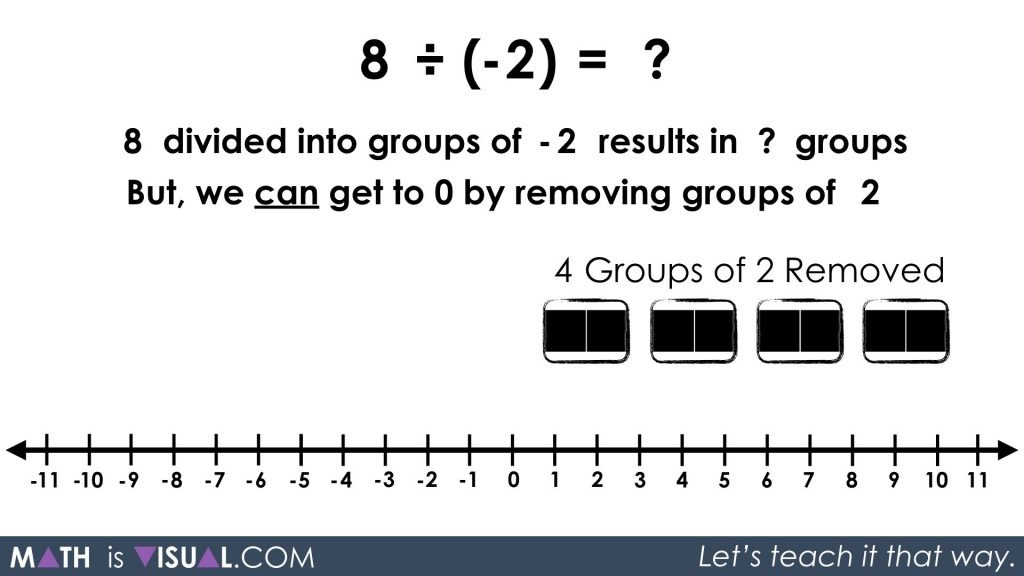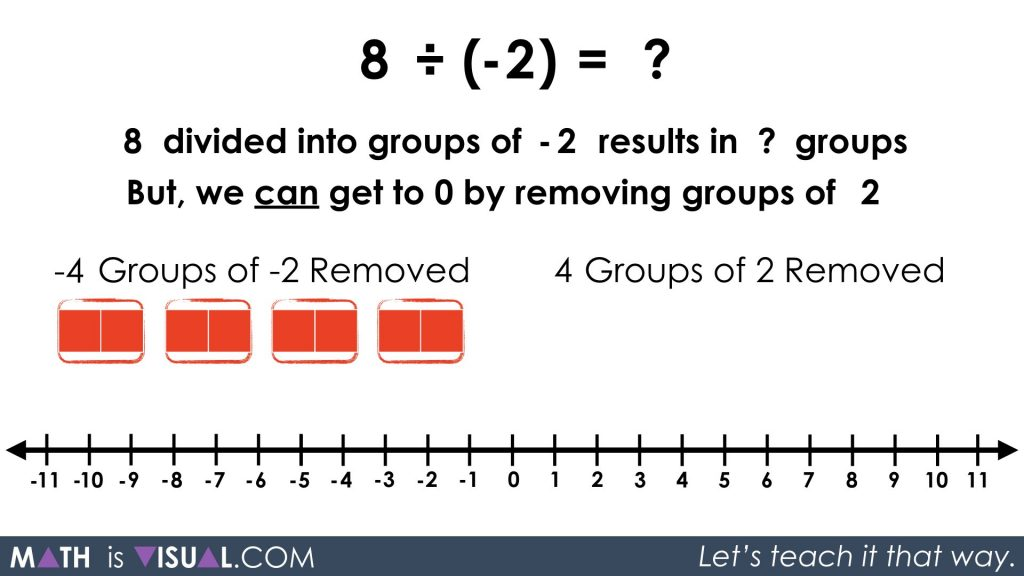We can also think about how many groups of -2 we can “add” to get to 0:Since repeated addition is the opposite operation of repeated subtraction, we can then take those groups and “subtract” them by negating the groupings:## Visual Prompt #3: Your Turn!

Pause the video and let the viewer apply their knowledge of positive number divided by a negative number using partitive division in the following visual prompt:

10 ÷ (-5) = ___

or

10 divided into -5 groups gives a result of ___ per group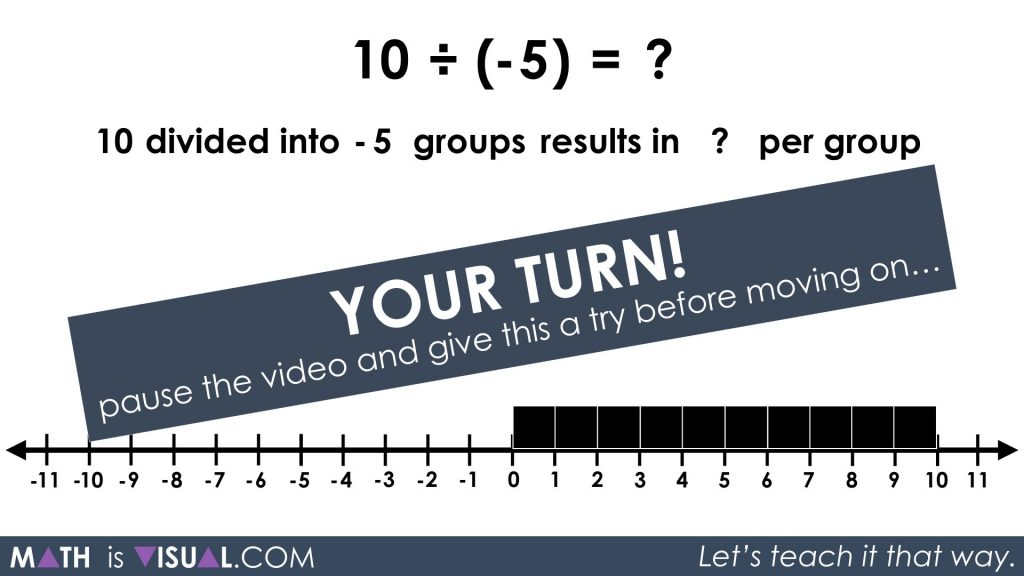Allow time for the viewer to engage in the partitive division before showing the remainder of the video animation or the following still image.

Again, we must note that we want to determine the value of the quantity that will be shared amongst 5 negatively charged groups or -5 groups.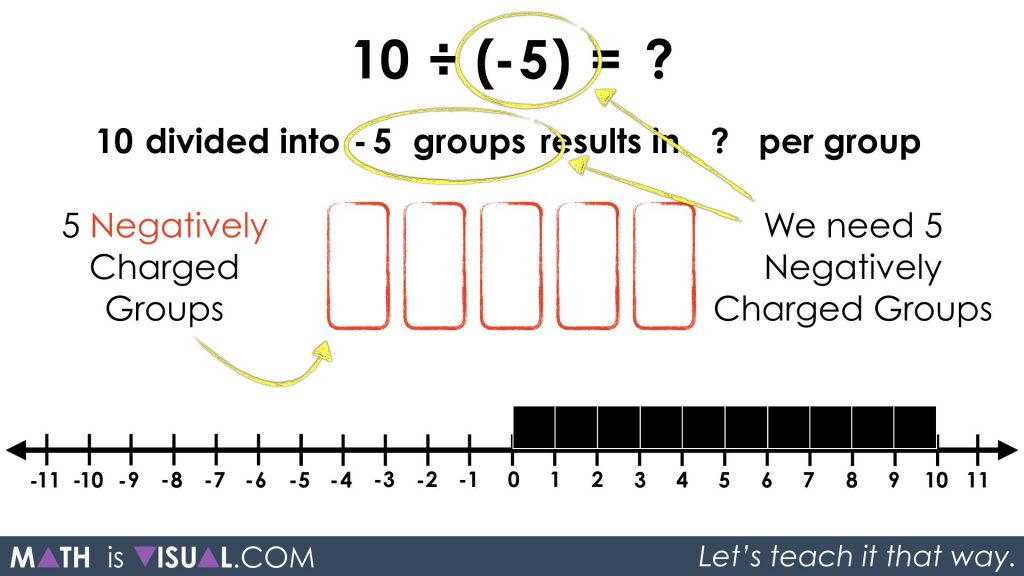By fair sharing 10 across the -5 groups, we see that each group will contain 2 negatives or -2.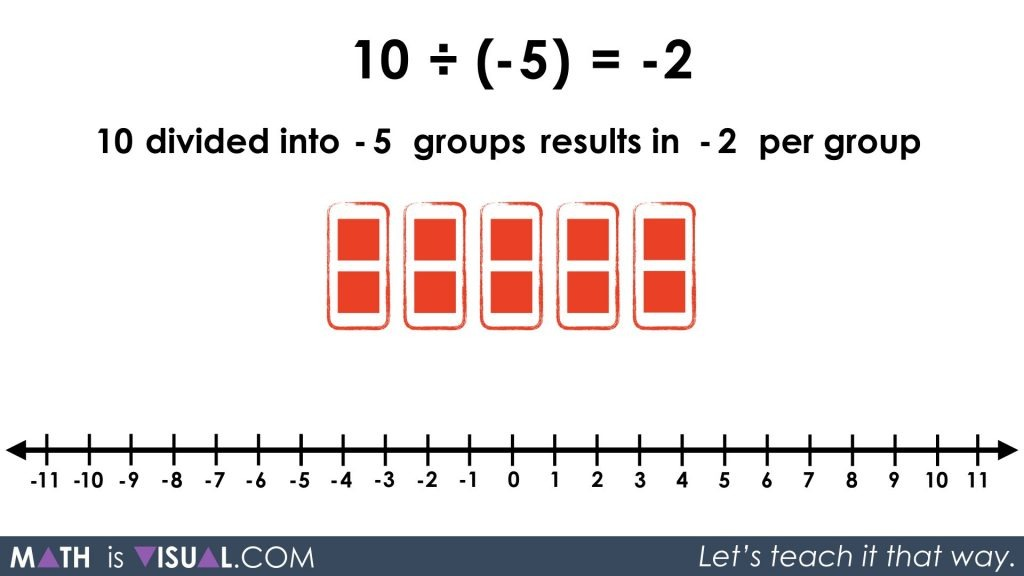How about approaching the same problem using quotative division:

10 ÷ (-5) = ___

or

10 divided into groups of -5 gives a result of ___ groups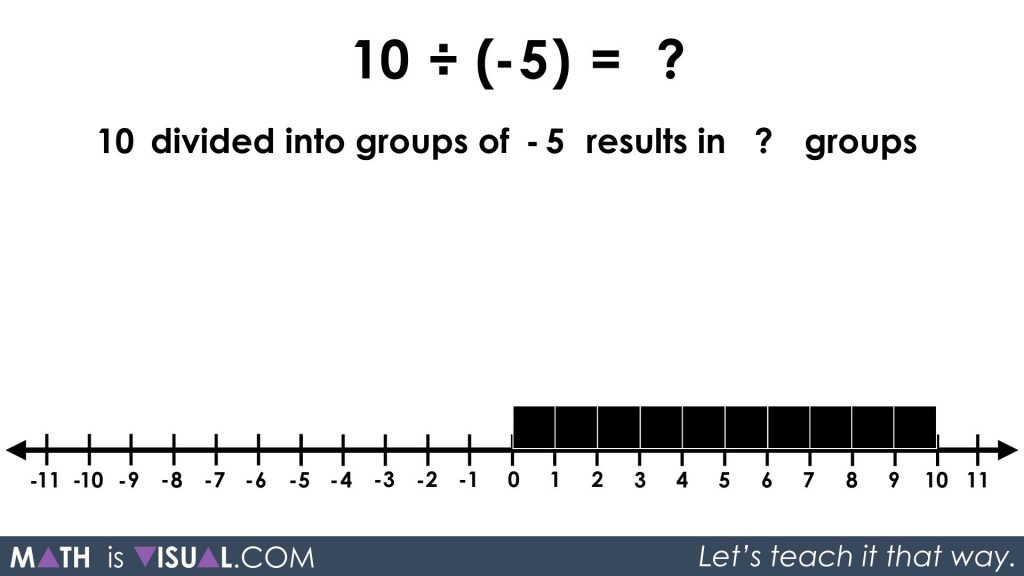By using our conceptual understanding built through the previous visual prompts, we can now recognize that since there are 0 groups of -5 we can create from the +10 given, we must use negatively charged groups.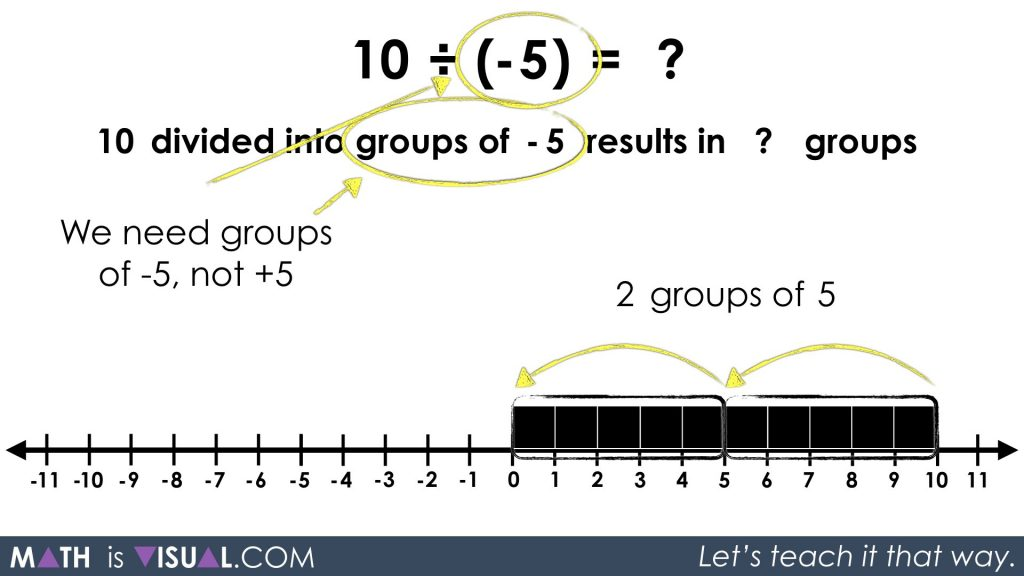Since I can create 2 groups of +5, I know I can also represent those same two groups as -2 groups of -5.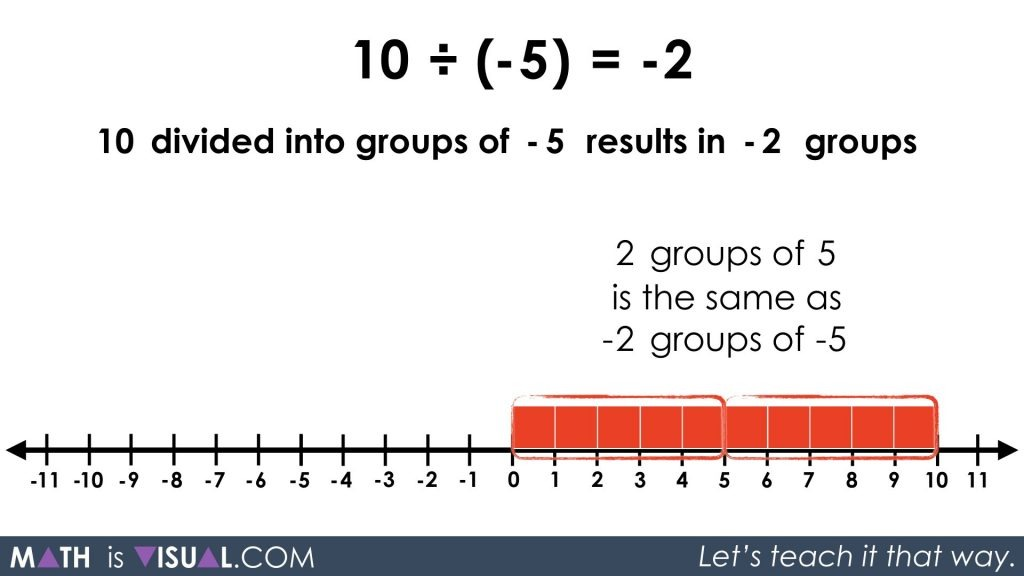There you have it! Understanding integer division conceptually through concrete and visual representations. In my classroom, I’d have students using coloured tiles or linking cubes to physically manipulate the materials to fully conceptualize this very important idea that often is approached using only rules and procedures.

Did you use this in your classroom or at home? How’d it go? Post in the comments!

Thanks for sticking around!

Math IS Visual. Let’s teach it that way.#### kylepearce3